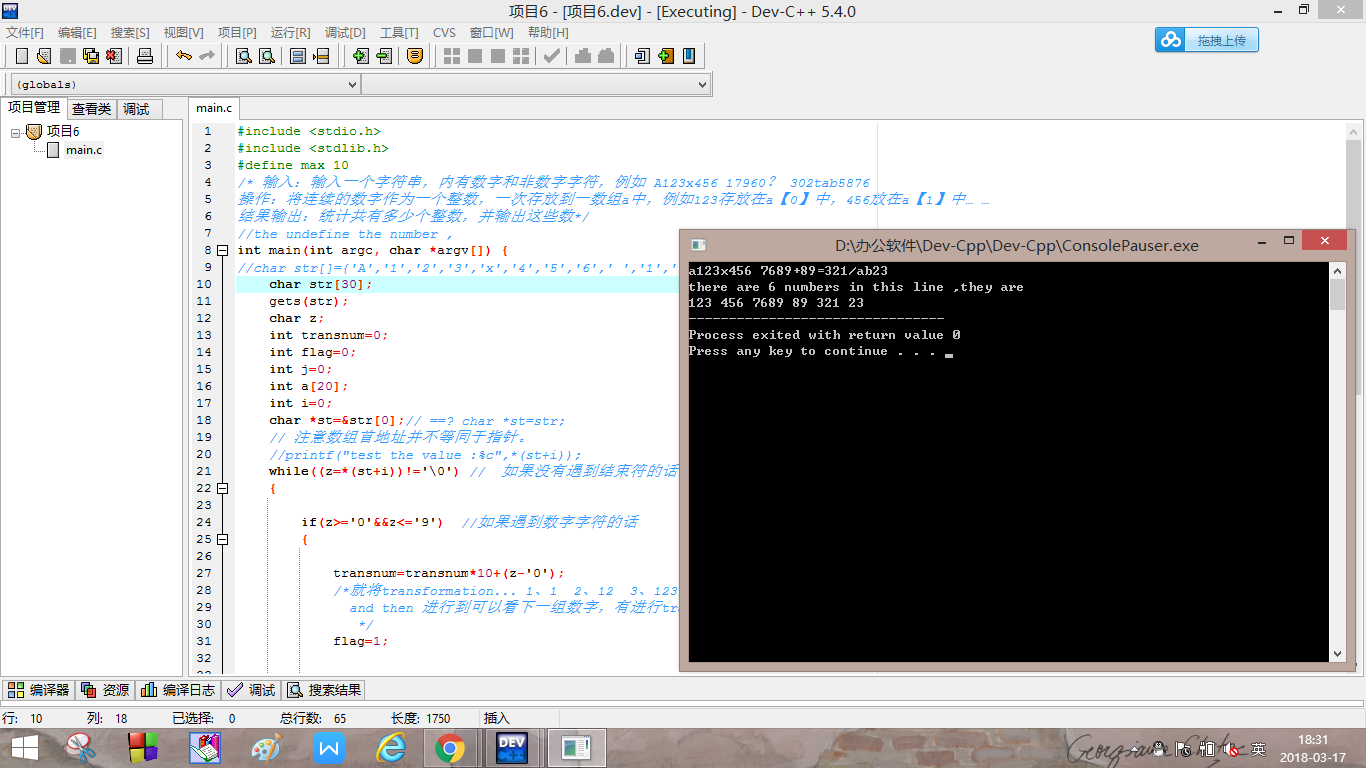# 输入一个字符串，内有数字和非数字字符，例如 A123x456 17960？ 302tab5876 操作：将连续的数字作为一个整数，一次存放到一数组a中，例如123存放在a【0】中，456放在a【1】中

#include <stdio.h>
#include <stdlib.h>
#define max 10
/* 输入：输入一个字符串，内有数字和非数字字符，例如 A123x456 17960？ 302tab5876

//the undefine the number ,
int main(int argc, char *argv[]) {
//char str[]={'A','1','2','3','x','4','5','6',' ','1','7','9','6','0','?','3','0','2','t','a','5','8','7','6','\0'};// total:25
char str;
gets(str);
char z;
int transnum=0;
int flag=0;
int j=0;
int a;
int i=0;
char *st=&str;// 等同于：char *st=str;
// 注意数组首地址并不等同于指针。
//printf("test the value :%c",*(st+i));
while((z=*(st+i))!='\0') //  如果没有遇到结束符的话
{

if(z>='0'&&z<='9')  //如果遇到数字字符的话
{

transnum=transnum*10+(z-'0'); //学会字符串转成数字的方法
/*就将transformation... 1、1  2、12  3、123 4、进行转存后然后就是i++ 操作，
and then 进行到可以看下一组数字，有进行transnum
*/
flag=1;

}
else

{
if(flag==1)   //遇到非数字的之后 保存前面的数字  flag=1 意思是前面是数字
{
a[j++]=transnum;
transnum=0;   // trannum 归为0，不然的话会造成123456 连续进行乘加法

}
flag=0;
}
i++;// 过一个字符就自增一次
}
if(flag==1)//最后结束符跳出了，但是数字还没有保存  flag=1 意思是前面是数字  所以while循环后保存数字到a[]数组中
{
a[j++]=transnum;
}
a[j]='\0';//注意最后加上'\0'，不然最后输出会有问题，当然也可以按照<j来输出，但是这样更加严谨
int *ast=a; //指针指向数组的首地址
printf("there are %d numbers in this line ,they are \n",j); //THE TOTAL NUMBER COUNT
i=0; //i归零
while(*(ast+i)!='\0')
{

printf("%d ",*(ast+i)); //指针指向输出
i++;  //勿忘i要进行自增
}

return 0;
}虽然思想不难，其中很多细节仍要处理清楚，很容易错误，我改了大约10处小错误。真的是可以的。不过也是印象深刻了，嘿嘿 。。